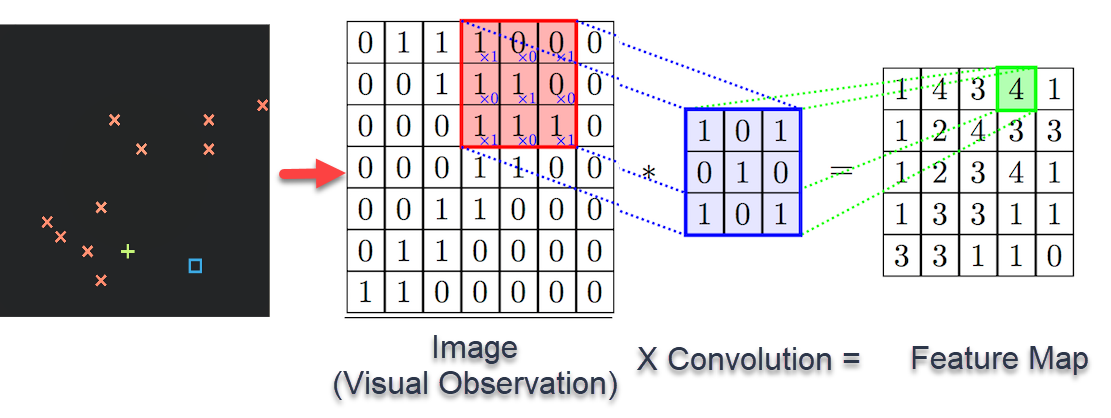### Computer vision: convolutional neural networks

When processing visual data, it seems that our technique of simply flattening the image and feeding it as a vector is a bit disappointing.

I mean, it works -- but remember what I said about the Bayesian prior? We should always try to build a network that is more inclined towards more likely models. We should make sure the network understands a priori that two pixels that are close to each other are more likely to interact to form a picture. But in turning images into vectors, all this information about proximity simply disappears.

What we want is a "hierarchial" notion of locality, where local features are studied, then local features of this feature map, and so on. In general, multiple features must be simultaneously analysed and combined in order for a larger goal, such as the identification of an image.

That multiple features must be simultaneously analysed for any non-trivial task should be completely obvious -- think, e.g. how you would produce any image analysis that depends on the gradient of the image or some other vector-valued quantity.

The biological motivation for this notion arises from the way that the brain perceives images, where each neuron only processes a portion of the visual data input known as the "receptive field", and these fields partially overlap between neurons.

So the idea is this: spread a little "box" of coefficients across your image, dotting the box with the local entries of your image and placing the results of the dot products in a new matrix. Like I said, we have a "vector" of boxes, so you get several matrix layers which can be treated as "feature channels" similar in essence to an RGB channel (that you might've actually had in the input image)(Source: https://subscription.packtpub.com/book/game_development/9781789138139/4/ch04lvl1sec31/convolutional-neural-networks)
In order to actually "capture" the local features and move on in our hierarchy, we then pool the feature values in the output map via little grids (making it much smaller). One may either think of pooling as testing for presence (max-pooling) or sustained presence (mean-pooling) of a feature. As the convolutions are done across the image, it generally isn't of fundamental importance which type of pooling we choose (at least when you have padding -- if you don't pad, max-pooling may be better).

To be more precise:
• Start with $(m,n)$ dimensional image with $p$ channels (e.g. RGB channels), and pad it with padding $(g, h)$ (to retain edge information).
• Rub $k$ filters on it of dimension $(a,b)$ ($p$ channels) with stride lengths $s,t$. These filters have weights as well as biases.
• You get a feature map of dimensions $\left(\left\lfloor\frac{m+2g-a}{s}\right\rfloor+1, \left\lfloor\frac{n+2h-b}{t}\right\rfloor+1\right)$ with $k$ channels.
• Pool with a pooling filter of dimension $(c, d)$ with stride lengths $u, v$ (should usually be equal to $c, d$). You get a pooled map of dimensions $\left(\left\lfloor\frac{\left\lfloor\frac{m+2g-a}{s}\right\rfloor+1-c}{u}\right\rfloor+1, \left\lfloor\frac{\left\lfloor\frac{n+2h-b}{t}\right\rfloor+1-d}{v}\right\rfloor+1\right)$ with $k$ channels.
• Feed into your activation function.
For the case $u=v=c=d$, $a=b$, $s=t=1$, $g=h$, the dimensions of the output are:

$$\left(\left\lfloor\frac{m + 2h - a +1}{u}\right\rfloor, \left\lfloor\frac{n + 2h - a +1}{u}\right\rfloor\right)$$

1. What does a larger filter (higher $a, b$) represent?